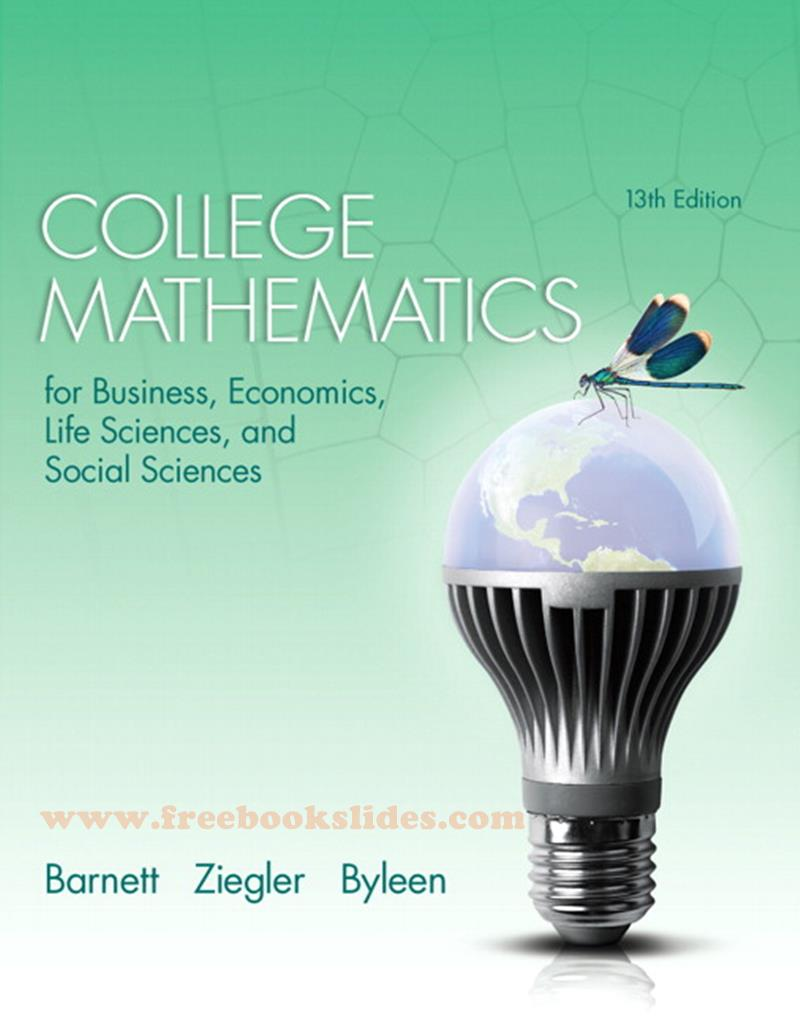### Ebook College Mathematics 13e by Barnett, Ziegler, ByleenCollege Mathematics for Business, Economics, Life Sciences, and Social Sciences
13th Edition
by Raymond A. Barnett • Michael R. Ziegler • Karl E. Byleen
Pe*rson Educ*tion / Pr*ntice Hall

PART ONE: A LIBRARY OF ELEMENTARY FUNCTIONS
1. Linear Equations and Graphs
2. Functions and Graphs

PART TWO: FINITE MATHEMATICS
3. Mathematics of Finance
4. Systems of Linear Equations; Matrices
5. Linear Inequalities and Linear Programming
6. Linear Programming: The Simplex Method
7. Logic, Sets, and Counting
8. Probability
9. Markov Chains

PART THREE: CALCULUS
10. Limits and the Derivative